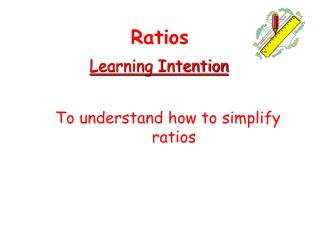DownloadDownload PresentationLearning Intention

# Learning Intention

Download Presentation## Learning Intention

- - - - - - - - - - - - - - - - - - - - - - - - - - - E N D - - - - - - - - - - - - - - - - - - - - - - - - - - -
##### Presentation Transcript

1. Ratios Learning Intention • To understand how to simplify ratios

2. ÷ 2 ÷ 2 Ratios Simplifying a ratio ? Ratios can be simplified like fractions by dividing each part by the same highest number. Highest Common Factor Fraction : = 6: 8 Ratio : 3 : 4

3. ÷ 3 ÷ 3 Ratios Simplifying a ratio ? For a three-part ratio all three parts must be divided by the same number. For example, 6 : 12 : 9 = 2 : 4 : 3

4. What is the ratio of red counters to blue counters? ÷ 3 ÷ 3 Ratios Simplifying a ratio ? For example, red : blue = 9 : 3 = 3 : 1 For every three red counters there is one blue counter.

5. For example, What is the ratio of blue counters to red counters? ÷ 3 ÷ 3 Ratios Simplifying a ratio ? The ratio of blue counters to red counters is not the same as the ratio of red counters to blue counters. blue : red = 3 : 9 = 1 : 3 For every blue counter there are three red counters.

6. What is the ratio of red counters to yellow counters to blue counters? ÷ 4 ÷ 4 Ratios Simplifying a ratio ? red : yellow : blue = 12 : 4 : 8 = 3 : 1 : 2 For every three red counters there is one yellow counter and two blue counters.

7. Ratios Simplifying a ratio ? Now try Ex 2 Ch15 Page 177